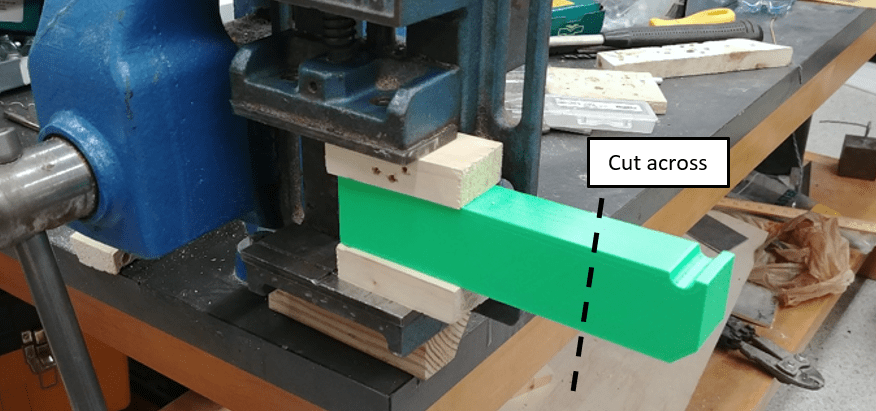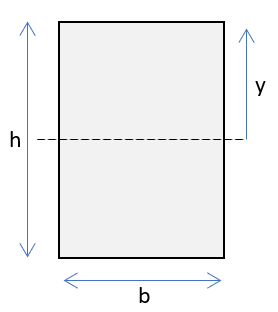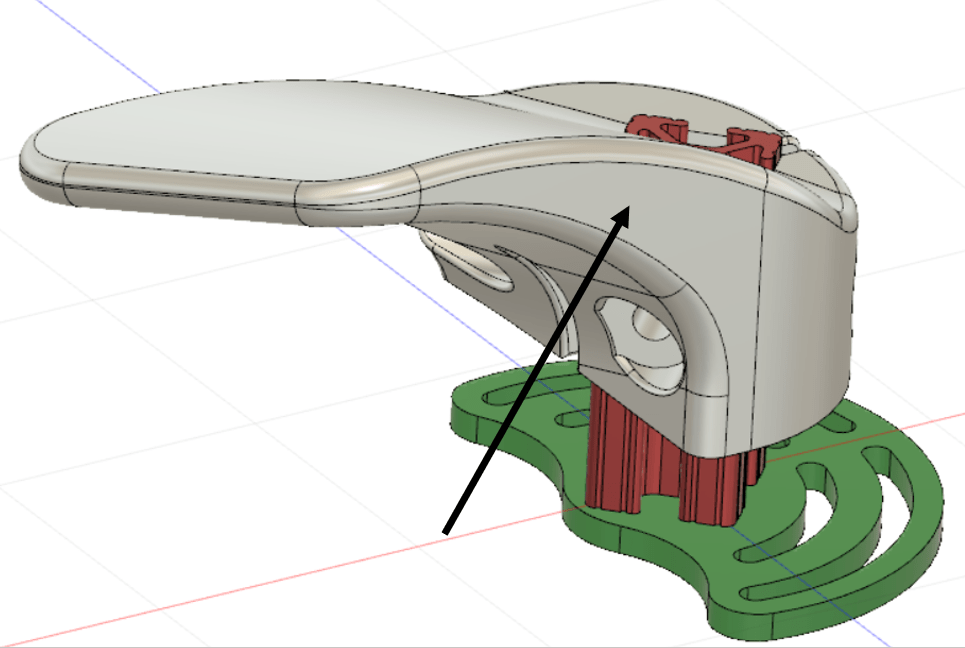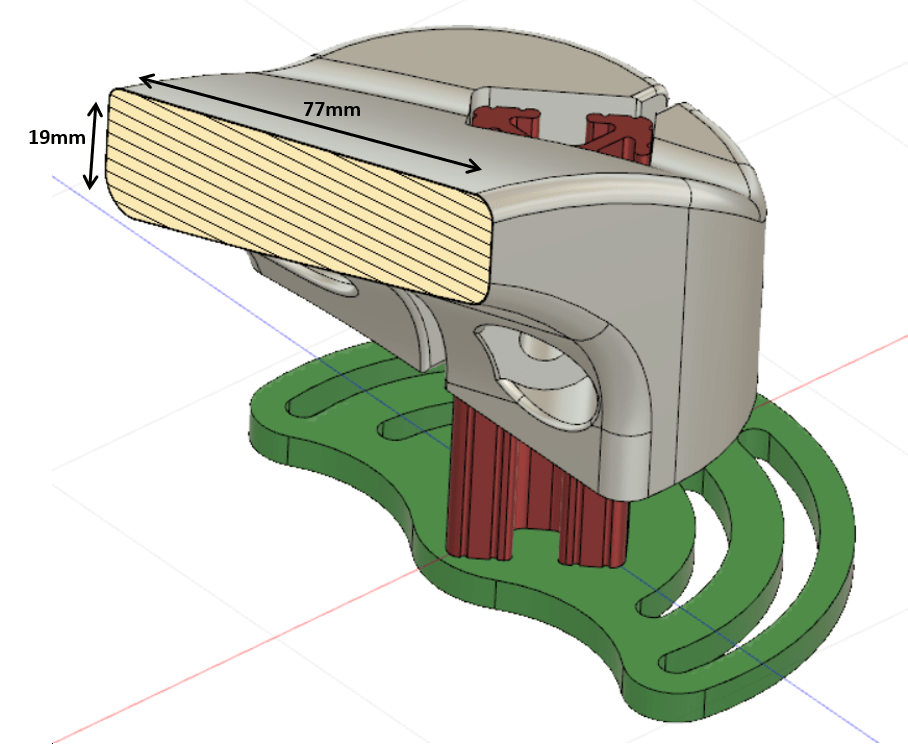Starting with rectangular beam in stresses is like writing "hello world" in coding.

It's time to finish your design!

A life fact first: we care more about the bending moment than the direct forces.

Just because moments kill stuff faster. (We can go into details in future posts, just let me know)

To calculate the bending stress we use a very simple formula:

S=M·y/I

"Stress equals bending moment (M) times offset (y) and divided by the moment of inertia (I)".
[Stress units are force divided by area]

All these are defined at the cross section.

# Cross Section?

This is the area you get when cutting your part/structure across.
Here is the green beam from the first post:Cutting along the beam

Because it's a rectangular beam, the cross section will be something like this:Rectangular cross section

# Moment of Inertia

And the moment of inertia will be:

I=h³·b/12

"The moment of inertia (I) equals the height (h) to the third power times width (b) divided by 12". Also, for this case only, y=h/2
[The units are length to the forth power]

This is true for rectangular area only. If you have another simple area, this can be easily calculated. For more complicated shapes, you can always approximate or use (almost all) CAD software packages.

Moment of inertia indicates the resistance to bending.

Larger value makes it harder to bend, and vise versa.
Also, taller cross sections are better than wider.

The moment you calculated last week, and if the dimensions are known, stress can be calculated.

But the reverse problem is much more interesting.

# Reverse Problem?

I'm 88kg, and I want to stand safely on a 130mm long clamped PLA beam.
What should be the dimensions of the cross section?

From here and here the failure stress of PLA is 3-4 kg/mm².

The goal is a rectangular beam with 30mm width (my starting point).

Shuffling things around and using y=h/2 we get something like this:

h²=6·M/b·S

Using 2 as a factor of safety (see post next week), h is ~39mm.

Rounding this up to 40mm finalizes the beam.

And it did hold me!

I believe this is better than printing stuff and hoping for it to hold.

What do you think?

# Recent example - 3d printed bindings

I introduced them last week, and you can make yourself a pair (or more) - Longboard Bindings

Let's calculate the stress around 45mm from the tip, right where the shape starts to curve. When applied 10kg at the tip, the bending moment at this point is ~500kg·mm. See previous post.

The cross section is curvy, but can be approximated as a rectangle.Right where the curve becomes even fatterYou can see kind of rectangle

Using the formula from before, the stress is around 0.1 kg/mm².

Definitely lower than 3 kg/mm² - the minimum failure stress of PLA.

Safe to use with even 10 times the load!

What do you think?

Is PLA a weak material and can't hold large loads?

What are you going to design next?

Enjoy,
Dani

Make sure to subscribe and get the next post in the series.

PS

I’m doing something new here.

A short series of posts dedicated to designing your own, as strong as you want, structures.

I’ll cover the basics, so you can do the rest.

Let me know what you think: dani@dosimplecarbon.com